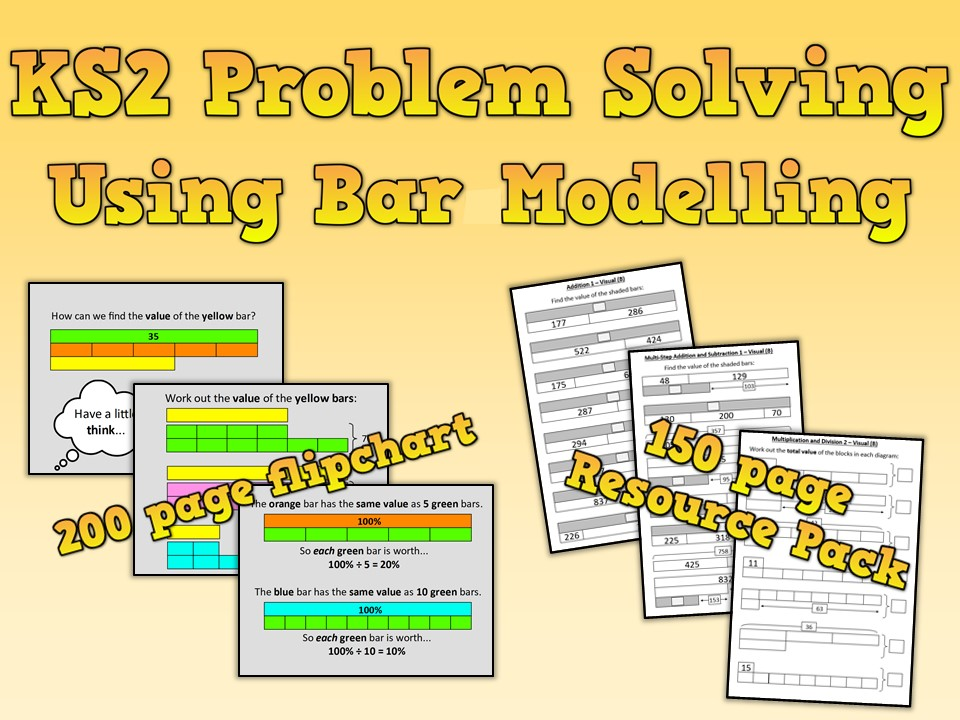I aim to produce complete resources, assessments and units of work that will save teachers many hours of planning, finding worksheets, making flip-charts, writing assessments etc. and to sell them at incredible prices. For more products visit www.mrlongsresources.com

Last updated

5 February 2020

#### Share thisflipchart, 6.43 MBpptx, 14.24 MBpdf, 14.06 MBpdf, 1.44 MBdocx, 1008.28 KB

This is a massive 150 page resource pack of visual and non-visual maths problems that use bar modelling to help visualise and solve maths problems (Singapore Maths). Each section has a collection of differentiated visual problems that, when practised, can then be used to solve the subsequent differentiated word problems. Also included is a MASSIVE 200 page flipchart (ActivInspire, PowerPoint and PDF formats) that is perfect for lesson introductions. In addition, the entire pack is available in Word format so that it can easily be edited for any year group or ability. Includes: Addition, subtraction, multiplication, division, fractions, ratio, percentages and algebra!

Objectives Covered

Solve problems, including missing number problems, using number facts, place value, and more complex addition and subtraction
Solve addition and subtraction two-step problems in contexts, deciding which operations and methods to use and why
Solve addition and subtraction multi-step problems in contexts, deciding which operations and methods to use and why
Solve problems, including missing number problems, involving multiplication and division, including positive integer scaling problems and correspondence problems in which n objects are connected to m object
Solve problems involving multiplying and adding, including using the distributive law to multiply two digit numbers by one digit, integer scaling problems and harder correspondence problems such as n objects are connected to m objects
Solve problems involving addition, subtraction, multiplication and division and a combination of these, including understanding the meaning of the equals sign
Solve problems involving multiplication and division, including scaling by simple fractions and problems involving simple rates
Solve problems involving addition, subtraction, multiplication and division
Solve problems involving increasingly harder fractions to calculate quantities, and fractions to divide quantities, including non-unit fractions where the answer is a whole number
Solve problems involving the relative sizes of two quantities where missing values can be found by using integer multiplication and division facts
Solve problems involving the calculation of percentages [for example, of measures, and such as 15% of 360] and use percentages for comparison
Solve problems involving similar shapes where the scale factor is known or can be found
Solve problems involving unequal sharing and grouping using knowledge of fractions and multiples

Visit my shop…

Visit My Shop!

### Reviews

5

Something went wrong, please try again later.

4 years ago
5

#### SimonRigelsford

4 years ago
5

great resource, well worth £7.99 for the time it will save me, thank you

#### Ktbattenti

4 years ago
5

Great resource, lots of examples for all different topics! Will be using it for SATs revision.

#### mandyfreeman

4 years ago
5

Hi, I have Smart board in my school. Is there any way you can send an iwb file for me to import to smart board? For some reason the export option is missing on my active inspire home software. Thanks in advance

#### helsinki123

5 years ago
5

These make perfect independent starter activities for my more able students. Thank you.

Report this resourceto let us know if it violates our terms and conditions.
Our customer service team will review your report and will be in touch.

£3.99